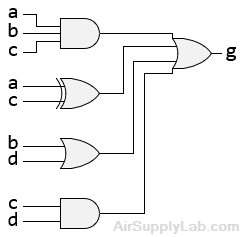# Lesson 07: Gate-Level (Structural) Modeling

Gate-Level Modeling

Gate-level modeling (also known as Structural modeling) can be used to write Verilog codes for small designs. Verilog HDL supports built-in primitive gates modeling. The gates supported are multiple-input, multiple-output, tristate, and pull gates. The multiple-input gates supported are: and, nand, or, nor, xor, and xnor whose number of inputs are two or more, and has only one output. The multiple-output gates supported are buf and not whose number of output is one or more, and has only one input. The language also supports the modeling of tri-state gates which include bufif0, bufif1, notif0, and notif1. These gates have one input, one control signal, and one output. The pull gates supported are pullup and pulldown with a single output (no input) only.

For gate-level modeling, the schematic for the combinational logic circuit must be drawn first.Figure 1: Schematic

The first step in using gate-level modeling is to check that all wires must have unique names. All the input/output wires have the same name as the I/O port name. In the schematic diagram of Figure 1, the output wires of the NOT and AND gates have no name, and they should be assigned a name. The wire name nc indicates the output wire of the NOT gate, and y0 indicates the output of the AND gate.

In the second step, use logic gates to describe the schematic from left-to-right, top-to-bottom.

Based on the schematic shown in Figure 1, the Verilog code can be written as follows:

module circuit1(a, b, c, f);
input  a, b, c;
output f;

wire  nc, y0;

not U1(nc, c);
and U2(y0, b, nc);
or  U3(f, y0, a);
endmodule

In the gate-level modeling, only the gates and wires can be used in the code.

The gate-level modeling is useful when a circuit is a simple combinational, as an example a multiplexer. Multiplexer is a simple circuit which connects one of many inputs to an output. In this part, you will create a simple 2-to-1 multiplexer and extend the design to multiple bits.

## Questions

Using gate-level (structural) modeling to solve the following questions.

1. Write the Verilog module to implement the circuit in the following figure using gate-level modeling.2. Write a Verilog description to implement the circuit of the following figure using gate-level modeling.